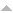## Publications

Export 76 results:
Sort by: Author Title [ Type] Year
Journal Article
Math. Proc. Cambridge Phil. Soc. , vol. 138, pp. 513-531, 2005.
SIAM journal on numerical analysis, vol. 47, issue 6, pp. 4711-4734 , 2010.
Math. Nachrichten, vol. 241, pp. 103-109 , 2002.
Theory Probab. Appl., vol. 53, pp. 216-241, 2009.
Math. Proc. Cambridge Phil. Soc., vol. 136, pp. 687-717, 2004.
Journal of Mathematical Analysis and Applications, vol. 318, no. 2, pp. 507 - 516, 2006.
Math. Nachrichten, vol. 183, pp. 113-133, 1997.
Mathematical Finance, vol. 15, no. 1, pp. 119-168, 2005.  Download: Quantization-Tree.pdf (483.24 KB)
Ann. Probab., vol. 30, pp. 874- 897, 2002.
Journal of Functional Analysis, vol. 212, no. 1, pp. 89 - 120, 2004.
J. Theor. Probab., vol. 16(2), pp. 427-449, 2003.
J. Comput. Appl. Math., vol. 89, no. 1, Amsterdam, The Netherlands, Elsevier Science Publishers B. V., pp. 1–38, 1998.
Monte Carlo Methods and Applications, vol. 7(1), pp. 21-34, 2001.
ESAIM: Probability and Statistics, vol. 13, pp. 218-246, 2009.
Miscellaneous
Numerical Methods in Finance, 2006.  Download: Pricing Path Dependent Option Using Functional Quantization.pdf (762.71 KB)
Thesis
Mathematik: Universität Trier, 2008.